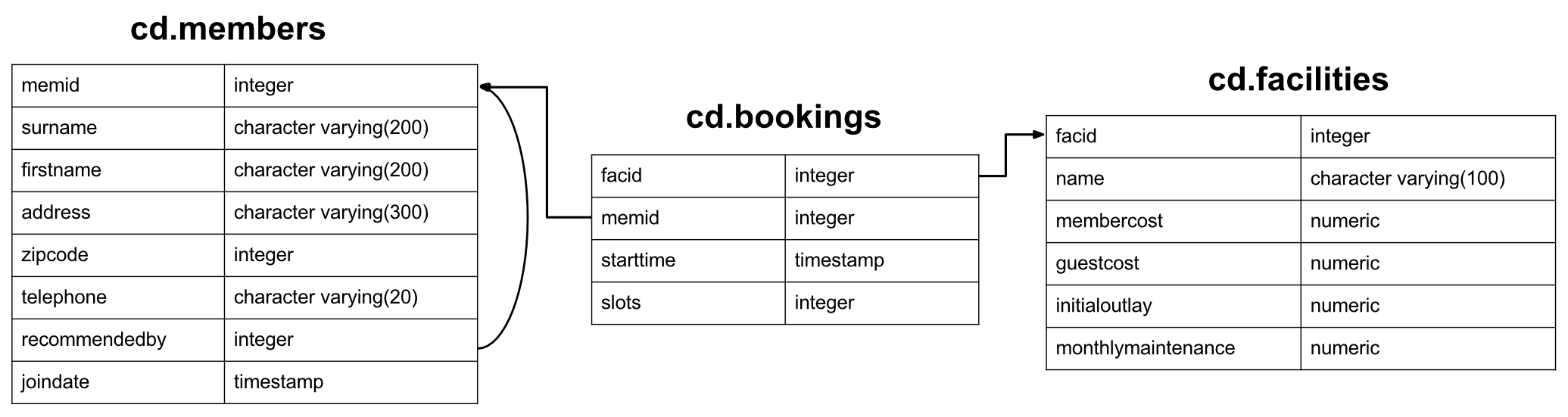### Question

Output the facility id that has the highest number of slots booked. Ensure that in the event of a tie, all tieing results get output.facid total
4 1404

### Your Answer```select facid, total from (
select facid, sum(slots) total, rank() over (order by sum(slots) desc) rank
from cd.bookings
group by facid
) as ranked
where rank = 1          ```

You may recall that this is a problem we've already solved in an earlier exercise. We came up with an answer something like below, which we then cut down using CTEs:

```select facid, sum(slots) as totalslots
from cd.bookings
group by facid
having sum(slots) = (select max(sum2.totalslots) from
(select sum(slots) as totalslots
from cd.bookings
group by facid
) as sum2);
```

Once we've cleaned it up, this solution is perfectly adequate. Explaining how the query works makes it seem a little odd, though - 'find the number of slots booked by the best facility. Calculate the total slots booked for each facility, and return only the rows where the slots booked are the same as for the best'. Wouldn't it be nicer to be able to say 'calculate the number of slots booked for each facility, rank them, and pick out any at rank 1'?

Fortunately, window functions allow us to do this - although it's fair to say that doing so is not trivial to the untrained eye. The first key piece of information is the existence of the RANK function. This ranks values based on the ORDER BY that is passed to it. If there's a tie for (say) second place), the next gets ranked at position 4. So, what we need to do is get the number of slots for each facility, rank them, and pick off the ones at the top rank. A first pass at this might look something like the below:

```select facid, total from (
select facid, total, rank() over (order by total desc) rank from (
select facid, sum(slots) total
from cd.bookings
group by facid
) as sumslots
) as ranked
where rank = 1
```

The inner query calculates the total slots booked, the middle one ranks them, and the outer one creams off the top ranked. We can actually tidy this up a little: recall that window function get applied pretty late in the select function, after aggregation. That being the case, we can move the aggregation into the ORDER BY part of the function, as shown in the approved answer.

While the window function approach isn't massively simpler in terms of lines of code, it arguably makes more semantic sense.

This one's a little bit tough. You'll need the RANK window function, and it's worth noting that it's possible to use an aggregate function inside the ORDER BY clause of a window function.